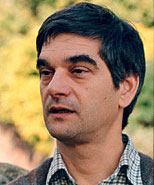# SNC 2014 Invited Speakers### Erich L. Kaltofen (North Carolina State University)

Algebraic error-correcting codes, such as the Reed-Solomon code, are based on polynomial interpolation with some erroneous values. The codes can be generalized to interpolation of sparse polynomials, which have fewer terms but for which the term exponents are unknown and the interpolation algorithms must compute them. A second generalization is to numerical interpolation where the values are imprecise and errors are viewed as statistical outliers.

We present sparse interpolation algorithms that can recover multivariate sparse polynomials and functions from approximate data with outliers. Our algorithms combine the exact sparse interpolation algorithms by Blahut and Zippel and their numeric counterparts by Giesbrecht-Labahn-Lee and Kaltofen-Yang-Zhi with the Welch-Berlekamp and Sudan error-correcting decoders and list-decoders. List-decoding allows for more errors and outliers while computing efficiently a list of interpolants that contains the original sparse function which was evaluated.

Our algorithms are doubly hybrid: they combine exact with numerical methods, in fact, constitute a numerical version of the algebraic error-correcting decoders, and recover both discrete outputs, the term degrees in the sparse support, and continuous data, the complex coefficients that approximately fit the data.

This is joint work with Clement Pernet [University of Grenoble] and Zhengfeng Yang [East China Normal University].### Lawrence Paulson (University of Cambridge)

MetiTarski is an automatic theorem prover for real-valued special functions. It proves formulas written in first-order logic concerning inequalities involving sin, cos, arctan, ln, exp, etc. MetiTarski has a novel architecture, combining a resolution theorem prover with external decision procedures for real-closed fields. Occurrences of special functions are replaced by rational function upper or lower bounds, ultimately reducing the original problem to a great many polynomial inequalities that can be solved using QEPCAD, Mathematica or Z3. The control structure of the theorem prover is a modified resolution loop incorporating case-splitting, as in SAT solvers.

Knowledge about the special functions is encapsulated in the form of axioms giving upper or lower bounds for each function over some suitable range. Some of these bounds are truncated Taylor series, while others arise from continued fraction approximations. A variety of these bounds, simple and complicated, with varying degrees of accuracy, are typically given to MetiTarski, whose heuristics automatically decide which ones to use in a proof.

Future developments will include providing ways to certify MetiTarski proofs and generalising it to handle wider range of applications. It also suggests new questions in approximation theory: hitherto, accuracy of the approximation has been the main concern, but the correctness of MetiTarski depends crucially on the use of approximations that are upper or lower bounds for a given function.### Bernd Sturmfels (University of California, Berkeley)

The nearest point map of a real algebraic variety with respect to Euclidean distance is an algebraic function. The Euclidean distance degree is the number of critical points of this optimization problem. We focus on varieties seen in engineering applications, and we discuss exact computational methods. Our running example is the Eckart-Young Theorem which states that the nearest point map for low rank matrices is given by the singular value decomposition. This is joint work with Jan Draisma, Emil Horobet, Giorgio Ottaviani, Rekha Thomas.

A preprint of the work is avalable at
http://front.math.ucdavis.edu/1309.0049.